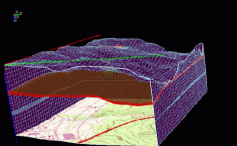## USC Component of PICS Activities: Analysis and Code Development

The USC component has focused its analytical work on the quantitative solution of the system of flow and transport equations, in particular, studies of possibly degenerate convection-diffusion equations. Degeneracy results from certain media properties which change nonlinearly with fluid saturation in the Richards approximation of the model equations.

Similarly, in two-phase flow, when the media becomes saturated with one fluid, the conservation equation of the remaining fluid tends to become degenerate. Due to numerical difficulties in numerically simulating these equations, a careful analysis of the regularizing approximation of these equations was carried out. In the total pressure-total velocity formulation of two-phase flow, the phase conservation equations are replaced by a total pressure equation and convection-diffusion water saturation equation.

In work joint with TAMU and BNL, USC personnel are investigating analytical and numerical aspects of both equations. The mixed finite element method is used to handle the pressure equation in order to preserve mass and to provide an accurate velocity field for the transport coupling and the determination of pressure. Numerical problems naturally arise in these saddle point problems due to the nonlinear tensors arising from the heterogeneous soil properties, as well as the nonlinear boundary conditions which arise from this formulation, and must be addressed through careful use of mathematical analysis. Multi-resolution techniques are also being analyzed in this context. In each case parallel implementation of the resulting algorithms is the major concern.

For convection-diffusion equations such as the saturation equation and multi-component transport equations, basic research is being conducted on Eulerian-Lagrangian Localized Ajoint Methods which were developed to address convection-dominated flows in a mass conservative manner. Additional advantages over other finite element methods in this setting include highly accurate and natural incorporation of boundary conditions, local refinement near fronts, and accurate domain decomposition. In this implementation test functions may be dynamically prescribed according to the underlying physics modeled by the partial differential equations.

Multiresolution analysis using biorthogonal wavelets and encoding algorithms are being used to investigate data compression and archival of large geophysical data sets that arise in connection with simulations. In collaboration with Ronald DeVore and an ONR project, we have developed wavelet compression techniques for multidimensional data sets which achieve high compression for use in Remote Tracking and Steering of simulations on remote supercomputers. Our initial work involved developing a 3D generalization of Shapiro's EZM encoder using 6-10 biorthogonal hyperbolic wavelets with appropriate extensions to handle logically rectangular data of arbitrary dimensions in the coordinate directions. In order to obtain higher compression rates and to make the transition from image compression to 3D (and higher dimensions) with arbitrary array sizes more transparent and efficient, we have developed new encoders which outperform current compression encoders even when restricted to the case of images.

Other potential uses of these multi-resolution methods are in the direction of 3D-feature extraction for registration in geophysical databases, denoising and assimilation of field data, and scaleup of parameter estimates from laboratory experiments. Wavelets are also being used to study nonlinear conservation laws and parabolic/elliptic Partial Differential Equations.

Sample model runs of the spread of a contaminant which were generated by PICS codes on USC's Paragon:

See also the related G3D visual rendering of the 1 million cells simulation (5.5 million unknowns) using 128 processors on ORNL's xps35 Paragon.

An animated transport simulation (with topomap overlay) is available with mpeg viewers (mpeg_play on UNIX workstations) by clicking here:Movie (approx. 2 MBytes)